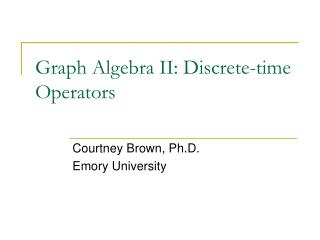DownloadDownload PresentationGraph Algebra II: Discrete-time Operators

# Graph Algebra II: Discrete-time Operators

Download Presentation## Graph Algebra II: Discrete-time Operators

- - - - - - - - - - - - - - - - - - - - - - - - - - - E N D - - - - - - - - - - - - - - - - - - - - - - - - - - -
##### Presentation Transcript

1. Graph Algebra II: Discrete-time Operators Courtney Brown, Ph.D. Emory University

2. The Delay and Advance Operators • E-1Input(t) = Input(t-1) = Output(t) • E1Input(t) = Input(t+1) = Output(t) • By convention, E1 is written simply as E, where superscripts are used only for higher-order advances. • These are linear operators that obey normal rules of algebra. • The operators work only on time-dependent variables. They do nothing to constants.

3. A Delay Operator in a Negative Feedback Loop

4. Getting the Equation • Using Mason’s Rule, V(t) = C(t)[p/(1+pmE-1)] • Thus, V(t)(1+pmE-1) = pC(t) V(t) + pmV(t)E-1 = pC(t) V(t) + pmV(t-1) = pC(t) • Now, multiply through by E to obtain EV(t) + pmEV(t-1) = pEC(t)

5. This gives us V(t+1) + pmV(t) = pC(t+1) • Re-arranging and adding an intercept constant, V(t+1) = pC(t+1) - pmV(t) + constant • This is a first-order linear difference equation with constant coefficients • In most of the social sciences, it is normal to have the lowest time subscript be t. Higher subscripts are t+_. • In economics, the convention is to write the highest time subscripts as t. Lower subscripts are t-_. • This is accomplished by multiplying through by either E or E-1 as needed.

6. Estimating the Model • The regression estimation equation would be V(t+1) = β0 + β1C(t+1) + β2V(t). where β0 = constant, β1 = p, and β2 = -pm • Once you have obtained β0, β1, and β2, you need to calculate the value of the parameters p and m. β1 and β2 are primarily of interest to you for this purpose. • However, the dynamic behavior of the model can nonetheless be deduced from the estimated values of β0, β1, and β2.

7. Including an Additive Constant • Here we include the constant k at the beginning of the feedback loop.

8. Another Approach to Including an Additive Constant • Here we include the additive constant after the beginning of the feedback loop.

9. Using a Difference Operator • In general, ΔY(t) = Y(t+1) – Y(t) • Why is this system over-determined?

10. The Discrete Summation Operator • Δ-1 acts to sum the flow of the path. • Δ and Δ-1 are inverse operations.

11. To show why Δ-1 sums the flow of a path, consider the simple statement Δ-1C(t)=V(t). • Since Δ-1 and Δ are inverse operations, we can also say that ΔV(t)=C(t), which is the same as saying V(t+1) – V(t) = C(t), or better still, V(t+1) = C(t) + V(t). • Now multiply through by E-1 to obtain, V(t) = C(t-1) + V(t-1) • Now substitute this for V(t) in the original equation at the top of this slide to obtainΔ-1C(t)= C(t-1) + V(t-1)

12. This demonstrates that the Δ-1 operator yields a mapping of an indefinite sum that results from having our current output equal to the addition of the input for the last time period and the output for the last time period. Thus, we are taking the previous input and adding it to the previous output to get the new output. With each new iteration, we are adding the one more input (from one time period only) to the previous output (which has been accumulating across all iterations), which is simply the accumulation of the input over time. (See also Cortez, Przeworski, and Sprague, pp. 299-300.)

13. Mixing Time Operators • Adding a time operator usually increases the order of the difference equation. • Try to avoid difference equations with orders higher than two.

14. Constants and Δ-1 • One of the basic techniques to remove a Δ-1 from a model is to multiply through by Δ. • But be careful if there is a constant in the model. Constants do not change, so when a constant bumps into a Δ, the product is 0. • For example, let us say your model is Yt+1 = a Δ-1Yt + b. Now, multiply through by Δ to obtain ΔYt+1 = aΔΔ-1Yt + Δb. But Δb=0, so we have ΔYt+1 = aYt.